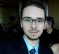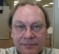## THD estimation: FFT vs PeriodogramStarted by 5 years ago7 replieslatest reply 5 years ago613 views

Hi all.

I think that maybe this question is too simple, but some explanation could give me a huge help.

I am working in an simple method review, witch aims to comply all methodology needed to acquire, filter and handle voltage data to estimate the THD (Total Harmonic Distortion).

Skipping the first acquisition process, assuming it was done in a right sampling frequency with enough ADC resolution, the next step is to apply some windowing to comply with periodicity requirements. Right after that, a zero padding is necessary to center the bean frequencies of the fft in the desirable frequencies. After that, a simple fft is done in matlab environment.

Taking the fft results, I normalize the amplitude by the length of non-zero (non-zero-padding) data. At this point, I have the abs of fft, with gives me the exactly voltage amplitude (of a simulated signal) in frequency spectrum.

Knowing the amplitude allows me to calculate the THD.

So, after all this, I refer to Matlab way of calculation. I see that Matlab uses a periodogram with a Kayser windown to estimate THD.

Knowing that my results are far better than Matlab THD function (at simulation), what is the difference to estimate data value over frequency spectrum using fft and using a periodogram?

Thanks.

[ - ]Maybe I'm missing something. I "thought" that a periodogram "was equivalent to" an FFT for "properly sampled data". So, periodogram should not enter into the discussion (I assume Matlab does it right). Sometimes there's a scaling issue for FFTs (there was/is for the DC component in MathCad). So if yours consistently differs from Matlab's result by a constant, then this is the issue, assuming you are using the EXACT SAME DATA SET FOR BOTH ANALYSES. Also, when you say "far better than" you mean your THD is lower, right? Again, check multiple examples and see if there's just a scaling issue.

So are you also using a Kaiser window on your data? If not, that would be a obvious difference, as windowing affects the amplitude and anything other than a square window (i.e. raw sampled data) results in frequency spread and its attendant amplitude reduction.

There are a few definitions of THD. First of all, THD is best done on a single frequency (or set of frequencies, one at a time). But this does not capture IM (intermodulation) distortion due to nonlinear operation.

The THD I am familiar with is the same was as Wikipedia explains it, except for their use of the term "RMS Voltage". In the past, I used the basic peak voltage as it comes out of an FFT (anyway, for basic sinusoids, that should not make a difference as the scaling cancels out, but only for basic sinusoids, not composite signals). If your sampled data has a lot of noise, or you are striving for super accurate THD (which is really hard in the real world because of noise for a single sample set), then you really need to use THD+N, unless you have LOTS of THD measurements so that the effects of "zero mean noise" (i.e. Gaussian) cancels out, since a Gaussian distribution is identical in the time-domain and frequency domains as the number of samples approaches infinity (or at least that what I recall from my research of several decades ago).

The hardest part of giving advice is not just the giver's knowledge, but also their interpretation of the problem and whether or not that includes all relevant details.

Implemented "correctly", implementations of any algorithm should always produce identical results, except for minor calculation noise.

[ - ]Maybe I'm missing something. I "thought" that a periodogram "was equivalent to" an FFT for "properly sampled data". So, periodogram should not enter into the discussion (I assume Matlab does it right). Sometimes there's a scaling issue for FFTs (there was/is for the DC component in MathCad). So if yours consistently differs from Matlab's result by a constant, then this is the issue, assuming you are using the EXACT SAME DATA SET FOR BOTH ANALYSES. Also, when you say "far better than" you mean your THD is lower, right? Again, check multiple examples and see if there's just a scaling issue.

When I said "far better than", I say that I have a calculated result (after all sampling and processing) much more closer with the real expected THD value. I only know the real THD value because I am generating the signal to this process, in the Matlab enviromment.

So are you also using a Kaiser window on your data? If not, that would be a obvious difference, as windowing affects the amplitude and anything other than a square window (i.e. raw sampled data) results in frequency spread and its attendant amplitude reduction.

Yes, I've used the same window (with the same characterization, provided in Matlab THD function description).

There are a few definitions of THD. First of all, THD is best done on a single frequency (or set of frequencies, one at a time). But this does not capture IM (intermodulation) distortion due to nonlinear operation.

Ok. What I am doing is to estimate the harmonic frequencies of a 60Hz signal, and calculating the total distortion of harmonics, relative to fundamental frequency. I also have inserted some other frequencies to test the robustness of the method. This other frequencies are located between harmonics.

The THD I am familiar with is the same was as Wikipedia explains it, except for their use of the term "RMS Voltage". In the past, I used the basic peak voltage as it comes out of an FFT (anyway, for basic sinusoids, that should not make a difference as the scaling cancels out, but only for basic sinusoids, not composite signals). If your sampled data has a lot of noise, or you are striving for super accurate THD (which is really hard in the real world because of noise for a single sample set), then you really need to use THD+N, unless you have LOTS of THD measurements so that the effects of "zero mean noise" (i.e. Gaussian) cancels out, since a Gaussian distribution is identical in the time-domain and frequency domains as the number of samples approaches infinity (or at least that what I recall from my research of several decades ago).

As I've explained above, there is only other frequencies, no noise at all. And, by sure, that frequencies are sampled according to nyquist rate, avoiding aliasing.

Implemented "correctly", implementations of any algorithm should always produce identical results, except for minor calculation noise.

I agree with that. I am using the same data set for both methods, trying to calculate all with same windows and functions, but the fft based method still giving me a different result than THD function.

There is any reason (statstically justified) to use an periodogram instead an fft for that kind of analysis? As you say too, wikipedia explains THD in therms of RMS voltage.. I really don't know how Matlab handle this.

[ - ]I do not use Matlab much so I may be wrong, but my first guess would be: Does Matlab mesaure THD+N instead of THD? That could make a huge difference depending on N...

[ - ]Hi,

I've explained above. Take a lookif you can, there is a better explanation of my problem now.

[ - ]Quote:

As I've explained above, there is only other frequencies, no noise at all. And, by sure, that frequencies are sampled according to nyquist rate, avoiding aliasing

These other frequencies will increase the THD+N value in comparison to the THD value. THD is sum of harmonics over the energy of the fundamental. THD+N is the the energy of everything except the fundamental over the energy of the fundamental. Everything except the fundamental includes non-harmonic frequencies.

[ - ]